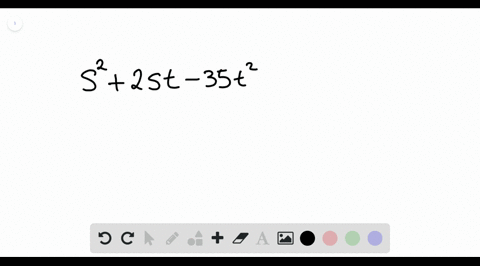Enroll in one of our FREE online STEM summer camps. Space is limited so join now!View Summer Courses### Factor each polynomial. If a polynomial cannot be…

01:17Other Schools

Need more help? Fill out this quick form to get professional live tutoring.

Get live tutoring
Problem 9

Factor each polynomial. If a polynomial cannot be factored, write prime. Factor out the greatest common factor as necessary.
$$a^{2}-6 a b+5 b^{2}$$

$a^{2}-6 a b+5 b^{2}=(a-b)(a-5 b)$

## Discussion

You must be signed in to discuss.

## Video Transcript

we're asked to factor this. Try no meal here. So the first term is a squared. Which means both factors must are with a the last term ISS five b squared, which means they must have something. Times be something times be So these two numbers here must multiply together to be positive five and add together to be negative six. And the only pair of numbers that can do that is negative. One comma negative, Fife. So this means you can fill those two numbers in. Now we get our factors are a minus one be. So it's which is just a minus. B an A minus five b.